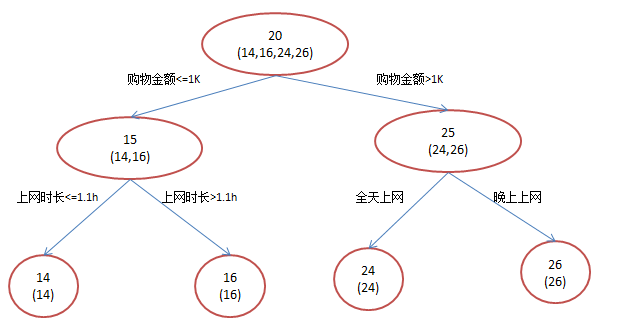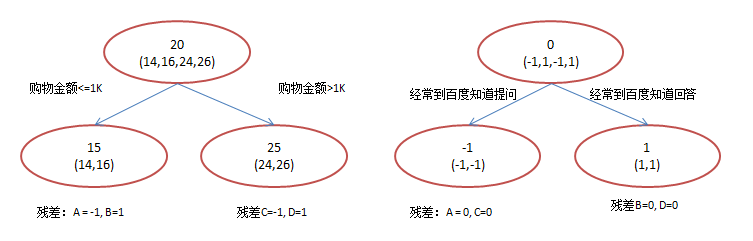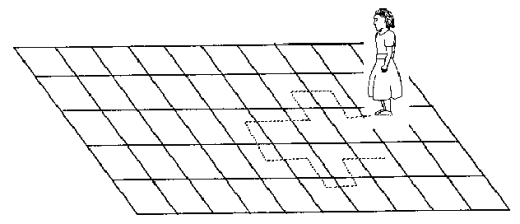## Python时间序列数据分析–以示例说明

### 导读

1. 用pandas处理时序数据
2. 怎样检查时序数据的稳定性
3. 怎样让时序数据具有稳定性
4. 时序数据的预测

### 1. 用pandas导入和处理时序数据

import pandas as pd
import numpy as np
import matplotlib.pylab as plt
from matplotlib.pylab import rcParams
#rcParams设定好画布的大小
rcParams['figure.figsize'] = 15, 6

http://github.com/aarshayj/Analytics_Vidhya/tree/master/Articles/Time_Series_Analysis 中下载

data = pd.read_csv(path+"AirPassengers.csv")
print '\n Data types:'
print data.dtypesdateparse = lambda dates: pd.datetime.strptime(dates, '%Y-%m')
#---其中parse_dates 表明选择数据中的哪个column作为date-time信息，
#---index_col 告诉pandas以哪个column作为 index
#--- date_parser 使用一个function(本文用lambda表达式代替)，使一个string转换为一个datetime变量
print data.index### 2.怎样检查时序数据的稳定性(Stationarity)

#### 1. 判断数据是稳定的常基于对于时间是常量的几个统计量：

1. 常量的均值
2. 常量的方差
3. 与时间独立的自协方差

1. 均值X是时序数据的值，t是时间。可以看到左图，数据的均值对于时间轴来说是常量，即数据的均值不是时间的函数,所有它是稳定的；右图随着时间的推移，数据的值整体趋势是增加的，所有均值是时间的函数，数据具有趋势，所以是非稳定的。
2. 方差可以看到左图，数据的方差对于时间是常量，即数据的值域围绕着均值上下波动的振幅是固定的，所以左图数据是稳定的。而右图，数据的振幅在不同时间点不同，所以方差对于时间不是独立的，数据是非稳定的。但是左、右图的均值是一致的。
3. 自协方差一个时序数据的自协方差，就是它在不同两个时刻i,j的值的协方差。可以看到左图的自协方差于时间无关；而右图，随着时间的不同，数据的波动频率明显不同，导致它i，j取值不同，就会得到不同的协方差，因此是非稳定的。虽然右图在均值和方差上都是与时间无关的，但仍是非稳定数据。

#### 2. python判断时序数据稳定性

1.Rolling statistic– 即每个时间段内的平均的数据均值和标准差情况。

1. Dickey-Fuller Test — 这个比较复杂，大致意思就是在一定置信水平下，对于时序数据假设 Null hypothesis: 非稳定。
if 通过检验值(statistic)< 临界值(critical value)，则拒绝null hypothesis，即数据是稳定的；反之则是非稳定的。
from statsmodels.tsa.stattools import adfuller
def test_stationarity(timeseries):

#这里以一年为一个窗口，每一个时间t的值由它前面12个月（包括自己）的均值代替，标准差同理。
rolmean = pd.rolling_mean(timeseries,window=12)
rolstd = pd.rolling_std(timeseries, window=12)

#plot rolling statistics:
fig = plt.figure()
orig = plt.plot(timeseries, color = 'blue',label='Original')
mean = plt.plot(rolmean , color = 'red',label = 'rolling mean')
std = plt.plot(rolstd, color = 'black', label= 'Rolling standard deviation')

plt.legend(loc = 'best')
plt.title('Rolling Mean & Standard Deviation')
plt.show(block=False)

#Dickey-Fuller test:

print 'Results of Dickey-Fuller Test:'
#dftest的输出前一项依次为检测值，p值，滞后数，使用的观测数，各个置信度下的临界值
dfoutput = pd.Series(dftest[0:4],index = ['Test Statistic','p-value','#Lags Used','Number of Observations Used'])
for key,value in dftest.items():
dfoutput['Critical value (%s)' %key] = value

print dfoutput

ts = data['#Passengers']
test_stationarity(ts)### 3. 让时序数据变成稳定的方法

1. 趋势（trend）-数据随着时间变化。比如说升高或者降低。
2. 季节性(seasonality)-数据在特定的时间段内变动。比如说节假日，或者活动导致数据的异常。

ts_log = np.log(ts)
1. 检测和去除趋势
通常有三种方法：

• 聚合 : 将时间轴缩短，以一段时间内星期/月/年的均值作为数据值。使不同时间段内的值差距缩小。
• 平滑： 以一个滑动窗口内的均值代替原来的值，为了使值之间的差距缩小
• 多项式过滤：用一个回归模型来拟合现有数据，使得数据更平滑。

Moving Average–移动平均

moving_avg = pd.rolling_mean(ts_log,12)
plt.plot(ts_log ,color = 'blue')
plt.plot(moving_avg, color='red')ts_log_moving_avg_diff = ts_log-moving_avg
ts_log_moving_avg_diff.dropna(inplace = True)
test_stationarity(ts_log_moving_avg_diff)# halflife的值决定了衰减因子alpha：  alpha = 1 - exp(log(0.5) / halflife)
expweighted_avg = pd.ewma(ts_log,halflife=12)
ts_log_ewma_diff = ts_log - expweighted_avg
test_stationarity(ts_log_ewma_diff)1. 检测和去除季节性
有两种方法：

• 1 差分化： 以特定滞后数目的时刻的值的作差
• 2 分解： 对趋势和季节性分别建模在移除它们

Differencing–差分

ts_log_diff = ts_log - ts_log.shift()
ts_log_diff.dropna(inplace=True)
test_stationarity(ts_log_diff)3.Decomposing-分解

#分解(decomposing) 可以用来把时序数据中的趋势和周期性数据都分离出来:
from statsmodels.tsa.seasonal import seasonal_decompose
def decompose(timeseries):

# 返回包含三个部分 trend（趋势部分） ， seasonal（季节性部分） 和residual (残留部分)
decomposition = seasonal_decompose(timeseries)

trend = decomposition.trend
seasonal = decomposition.seasonal
residual = decomposition.resid

plt.subplot(411)
plt.plot(ts_log, label='Original')
plt.legend(loc='best')
plt.subplot(412)
plt.plot(trend, label='Trend')
plt.legend(loc='best')
plt.subplot(413)
plt.plot(seasonal,label='Seasonality')
plt.legend(loc='best')
plt.subplot(414)
plt.plot(residual, label='Residuals')
plt.legend(loc='best')
plt.tight_layout()

return trend , seasonal, residual#消除了trend 和seasonal之后，只对residual部分作为想要的时序数据进行处理
trend , seasonal, residual = decompose(ts_log)
residual.dropna(inplace=True)
test_stationarity(residual)### 4. 对时序数据进行预测

step1： 通过ACF,PACF进行ARIMA（p，d，q）的p，q参数估计

yt=YtYt1yt=Yt−Yt−1

#ACF and PACF plots:
from statsmodels.tsa.stattools import acf, pacf
lag_acf = acf(ts_log_diff, nlags=20)
lag_pacf = pacf(ts_log_diff, nlags=20, method='ols')
#Plot ACF:
plt.subplot(121)
plt.plot(lag_acf)
plt.axhline(y=0,linestyle='--',color='gray')
plt.axhline(y=-1.96/np.sqrt(len(ts_log_diff)),linestyle='--',color='gray')
plt.axhline(y=1.96/np.sqrt(len(ts_log_diff)),linestyle='--',color='gray')
plt.title('Autocorrelation Function')

#Plot PACF:
plt.subplot(122)
plt.plot(lag_pacf)
plt.axhline(y=0,linestyle='--',color='gray')
plt.axhline(y=-1.96/np.sqrt(len(ts_log_diff)),linestyle='--',color='gray')
plt.axhline(y=1.96/np.sqrt(len(ts_log_diff)),linestyle='--',color='gray')
plt.title('Partial Autocorrelation Function')
plt.tight_layout()step2： 得到参数估计值p，d，q之后，生成模型ARIMA（p，d，q）

from statsmodels.tsa.arima_model import ARIMA
model = ARIMA(ts_log, order=(2, 1, 0))
results_AR = model.fit(disp=-1)
plt.plot(ts_log_diff)
plt.plot(results_AR.fittedvalues, color='red')
plt.title('RSS: %.4f'% sum((results_AR.fittedvalues-ts_log_diff)**2))model = ARIMA(ts_log, order=(0, 1, 2))
results_MA = model.fit(disp=-1)
plt.plot(ts_log_diff)
plt.plot(results_MA.fittedvalues, color='red')
plt.title('RSS: %.4f'% sum((results_MA.fittedvalues-ts_log_diff)**2))model = ARIMA(ts_log, order=(2, 1, 2))
results_ARIMA = model.fit(disp=-1)
plt.plot(ts_log_diff)
plt.plot(results_ARIMA.fittedvalues, color='red')
plt.title('RSS: %.4f'% sum((results_ARIMA.fittedvalues-ts_log_diff)**2))step3: 将模型代入原数据进行预测


#ARIMA拟合的其实是一阶差分ts_log_diff，predictions_ARIMA_diff[i]是第i个月与i-1个月的ts_log的差值。
#由于差分化有一阶滞后，所以第一个月的数据是空的，
predictions_ARIMA_diff = pd.Series(results_ARIMA.fittedvalues, copy=True)
#累加现有的diff，得到每个值与第一个月的差分（同log底的情况下）。
#即predictions_ARIMA_diff_cumsum[i] 是第i个月与第1个月的ts_log的差值。
predictions_ARIMA_diff_cumsum = predictions_ARIMA_diff.cumsum()
#先ts_log_diff => ts_log=>ts_log => ts
#先以ts_log的第一个值作为基数，复制给所有值，然后每个时刻的值累加与第一个月对应的差值(这样就解决了，第一个月diff数据为空的问题了)
#然后得到了predictions_ARIMA_log => predictions_ARIMA
predictions_ARIMA_log = pd.Series(ts_log.ix, index=ts_log.index)
predictions_ARIMA = np.exp(predictions_ARIMA_log)
plt.figure()
plt.plot(ts)
plt.plot(predictions_ARIMA)
plt.title('RMSE: %.4f'% np.sqrt(sum((predictions_ARIMA-ts)**2)/len(ts)))### 5.总结

(1). 获取被观测系统时间序列数据；
(2). 对数据绘图，观测是否为平稳时间序列；对于非平稳时间序列要先进行d阶差分运算，化为平稳时间序列；
(3). 经过第二步处理，已经得到平稳时间序列。要对平稳时间序列分别求得其自相关系数ACF 和偏自相关系数PACF，通过对自相关图和偏自相关图的分析，得到最佳的阶层 p 和阶数 q
(4). 由以上得到的d、q、p，得到ARIMA模型。然后开始对得到的模型进行模型检验。

1.判断一个时序数据是否是稳定。对应步骤(1)

1. 怎样让时序数据稳定化。对应步骤(2)
2. 使用ARIMA模型进行时序数据预测。对应步骤(3,4)

https://www.analyticsvidhya.com/blog/

## GBDT（MART） 迭代决策树入门教程 | 简介

2012年11月29日 19:12:19

【1】Boosting Decision Tree入门教程 http://www.schonlau.net/publication/05stata_boosting.pdf

【2】LambdaMART用于搜索排序入门教程 http://research.microsoft.com/pubs/132652/MSR-TR-2010-82.pdfA: 14岁高一学生，购物较少，经常问学长问题；预测年龄A = 15 – 1 = 14

B: 16岁高三学生；购物较少，经常被学弟问问题；预测年龄B = 15 + 1 = 16

C: 24岁应届毕业生；购物较多，经常问师兄问题；预测年龄C = 25 – 1 = 24

D: 26岁工作两年员工；购物较多，经常被师弟问问题；预测年龄D = 25 + 1 = 26

1）既然图1和图2 最终效果相同，为何还需要GBDT呢？

Shrinkage（缩减）的思想认为，每次走一小步逐渐逼近结果的效果，要比每次迈一大步很快逼近结果的方式更容易避免过拟合。即它不完全信任每一个棵残差树，它认为每棵树只学到了真理的一小部分，累加的时候只累加一小部分，通过多学几棵树弥补不足。用方程来看更清晰，即

y(i+1) = 残差(y1~yi)， 其中： 残差(y1~yi) =  y真实值 – y(1 ~ i)

y(1 ~ i) = SUM(y1, …, yi)

Shrinkage不改变第一个方程，只把第二个方程改为：

y(1 ~ i) = y(1 ~ i-1) + step * yi

# 引言

Boosting 分类器属于集成学习模型，它基本思想是把成百上千个分类准确率较低的树模型组合起来，成为一个准确率很高的模型。这个模型会不断地迭代，每次迭代就生成一颗新的树。对于如何在每一步生成合理的树，大家提出了很多的方法，我们这里简要介绍由 Friedman 提出的 Gradient Boosting Machine。它在生成每一棵树的时候采用梯度下降的思想，以之前生成的所有树为基础，向着最小化给定目标函数的方向多走一步。在合理的参数设置下，我们往往要生成一定数量的树才能达到令人满意的准确率。在数据集较大较复杂的时候，我们可能需要几千次迭代运算，如果生成一个树模型需要几秒钟，那么这么多迭代的运算耗时，应该能让你专心地想静静……# 功能介绍

## 一、基础功能

devtools::install_github('dmlc/xgboost',subdir='R-package')


require(xgboost)
data(agaricus.train, package='xgboost')
data(agaricus.test, package='xgboost')
train <- agaricus.train
test <- agaricus.test


class(train$data)   "dgCMatrix"  attr(,"package")   "Matrix"  不用担心，xgboost 支持稀疏矩阵作为输入。下面就是训练模型的命令 bst <- xgboost(data = train$data, label = train$label, max.depth = 2, eta = 1,nround = 2, objective = "binary:logistic")   train-error:0.046522  train-error:0.022263  我们迭代了两次，可以看到函数输出了每一次迭代模型的误差信息。这里的数据是稀疏矩阵，当然也支持普通的稠密矩阵。如果数据文件太大不希望读进 R 中，我们也可以通过设置参数data = 'path_to_file'使其直接从硬盘读取数据并分析。目前支持直接从硬盘读取 libsvm 格式的文件。 做预测只需要一句话： pred <- predict(bst, test$data)


cv.res <- xgb.cv(data = train$data, label = train$label, max.depth = 2, eta = 1, nround = 2, objective = "binary:logistic",
nfold = 5)

 train-error:0.046522+0.001102   test-error:0.046523+0.004410
 train-error:0.022264+0.000864   test-error:0.022266+0.003450

cv.res

   train.error.mean train.error.std test.error.mean test.error.std
1:         0.046522        0.001102        0.046523       0.004410
2:         0.022264        0.000864        0.022266       0.003450


## 二、高速准确

1. xgboost 借助 OpenMP，能自动利用单机 CPU 的多核进行并行计算。需要注意的是，Mac 上的 Clang 对 OpenMP 的支持较差，所以默认情况下只能单核运行。
2. xgboost 自定义了一个数据矩阵类 DMatrix，会在训练开始时进行一遍预处理，从而提高之后每次迭代的效率。

Time (in secs) 761.48 450.22 102.41 44.18 34.04

## 三、进阶特征

1. 只要能够求出目标函数的梯度和 Hessian 矩阵，用户就可以自定义训练模型时的目标函数。demo
2. 允许用户在交叉验证时自定义误差衡量方法，例如回归中使用 RMSE 还是 RMSLE，分类中使用 AUC，分类错误率或是 F1-score。甚至是在希格斯子比赛中的 “奇葩” 衡量标准 AMSdemo
3. 交叉验证时可以返回模型在每一折作为预测集时的预测结果，方便构建 ensemble 模型。demo
4. 允许用户先迭代 1000 次，查看此时模型的预测效果，然后继续迭代 1000 次，最后模型等价于一次性迭代 2000 次。demo
6. 可以计算变量重要性并画出树状图。demo
7. 可以选择使用线性模型替代树模型，从而得到带 L1+L2 惩罚的线性回归或者 logistic 回归。demo

# 结语

xgboost 功能较多，参数设置比较繁杂，希望在上手之后有更全面了解的读者可以参考项目 wiki。欢迎大家多多交流，在项目 issue 区提出疑问与建议。我们也邀请有兴趣的读者提交代码完善功能，让 xgboost 成为更好用的工具。

## 什么是 ARIMA模型

ARIMA模型的全称叫做自回归移动平均模型，全称是(ARIMA, Autoregressive Integrated Moving Average Model)。也记作ARIMA(p,d,q)，是统计模型(statistic model)中最常见的一种用来进行时间序列 预测的模型。

### 1. ARIMA的优缺点

1.要求时序数据是稳定的（stationary），或者是通过差分化(differencing)后是稳定的。
2.本质上只能捕捉线性关系，而不能捕捉非线性关系。

### 2. 判断是时序数据是稳定的方法。

1. 稳定的数据是没有趋势(trend)，没有周期性(seasonality)的; 即它的均值，在时间轴上拥有常量的振幅，并且它的方差，在时间轴上是趋于同一个稳定的值的。
2. 可以使用Dickey-Fuller Test进行假设检验。（另起文章介绍）

### 3. ARIMA的参数与数学形式

ARIMA模型有三个参数:p,d,q。

• p–代表预测模型中采用的时序数据本身的滞后数(lags) ,也叫做AR/Auto-Regressive项
• d–代表时序数据需要进行几阶差分化，才是稳定的，也叫Integrated项。
• q–代表预测模型中采用的预测误差的滞后数(lags)，也叫做MA/Moving Average项

if d=0, yt=Ytif d=1, yt=YtYt1if d=2, yt=(YtYt1)(Yt1Yt2)=Yt2Yt1+Yt2if d=0, yt=Ytif d=1, yt=Yt−Yt−1if d=2, yt=(Yt−Yt−1)−(Yt−1−Yt−2)=Yt−2Yt−1+Yt−2

ARIMA的预测模型可以表示为：

Y的预测值 = 常量c and/or 一个或多个最近时间的Y的加权和 and/or 一个或多个最近时间的预测误差。

ARIMA用数学形式表示为：

ytˆ=μ+ϕ1yt1+...+ϕpytp+θ1et1+...+θqetqyt^=μ+ϕ1∗yt−1+…+ϕp∗yt−p+θ1∗et−1+…+θq∗et−q

,ϕARθMA其中,ϕ表示AR的系数，θ表示MA的系数

### 4.ARIMA模型的几个特例

#### 1.ARIMA(0,1,0) = random walk:Yˆt=μ+Yt1Y^t=μ+Yt−1

#### 2. ARIMA(1,0,0) = first-order autoregressive model:

p=1, d=0,q=0。说明时序数据是稳定的和自相关的。一个时刻的Y值只与上一个时刻的Y值有关。

Yˆt=μ+ϕ1Yt1.where, ϕ[1,1],Y^t=μ+ϕ1∗Yt−1.where, ϕ∈[−1,1],是一个斜率系数

#### 3. ARIMA(1,1,0) = differenced first-order autoregressive model:

p=1,d=1,q=0. 说明时序数据在一阶差分化之后是稳定的和自回归的。即一个时刻的差分（y）只与上一个时刻的差分有关。

yˆt=μ+ϕ1yt1YˆtYt1=μ+ϕ1(Yt1Yt2)Yˆt=μ+Yt1+ϕ1(Yt1Yt2)y^t=μ+ϕ1∗yt−1结合一阶差分的定义，也可以表示为：Y^t−Yt−1=μ+ϕ1∗(Yt−1−Yt−2)或者Y^t=μ+Yt−1+ϕ1∗(Yt−1−Yt−2)

#### 4. ARIMA(0,1,1) = simple exponential smoothing with growth.

p=0, d=1 ,q=1.说明数据在一阶差分后市稳定的和移动平均的。即一个时刻的估计值的差分与上一个时刻的预测误差有关。

yˆt=μ+α1et1q=1ytp=1ytyˆt=YˆtYˆt1, et1=Yt1Yˆt1,θ1=1α1Yˆt=μ+Yˆt1+α1(Yt1Yˆt1)=μ+Yt1θ1et1y^t=μ+α1∗et−1注意q=1的差分yt与p=1的差分yt的是不一样的其中，y^t=Y^t−Y^t−1, et−1=Yt−1−Y^t−1,设θ1=1−α1则也可以写成：Y^t=μ+Y^t−1+α1(Yt−1−Y^t−1)=μ+Yt−1−θ1∗et−1

#### 5. ARIMA(2,1,2)

yˆt=μ+ϕ1yt1+ϕ2yt2θ1et1θ2et2:Yˆt=μ+ϕ1(Yt1Yt2)+ϕ2(Yt2Yt3)θ1(Yt1Yˆt1)θ2(Yt2Yˆt2)y^t=μ+ϕ1∗yt−1+ϕ2∗yt−2−θ1∗et−1−θ2∗et−2也可以写成:Y^t=μ+ϕ1∗(Yt−1−Yt−2)+ϕ2∗(Yt−2−Yt−3)−θ1∗(Yt−1−Y^t−1)−θ2∗(Yt−2−Y^t−2)

#### 6. ARIMA(2,2,2)

yˆt=μ+ϕ1yt1+ϕ2yt2θ1et1θ2et2Yˆt=μ+ϕ1(Yt12Yt2+Yt3)+ϕ2(Yt22Yt3+Yt4)θ1(Yt1Yˆt1)θ2(Yt2Yˆt2)y^t=μ+ϕ1∗yt−1+ϕ2∗yt−2−θ1∗et−1−θ2∗et−2Y^t=μ+ϕ1∗(Yt−1−2Yt−2+Yt−3)+ϕ2∗(Yt−2−2Yt−3+Yt−4)−θ1∗(Yt−1−Y^t−1)−θ2∗(Yt−2−Y^t−2)

#### 7. ARIMA建模基本步骤

1. 获取被观测系统时间序列数据；
2. 对数据绘图，观测是否为平稳时间序列；对于非平稳时间序列要先进行d阶差分运算，化为平稳时间序列；
3. 经过第二步处理，已经得到平稳时间序列。要对平稳时间序列分别求得其自相关系数ACF 和偏自相关系数PACF，通过对自相关图和偏自相关图的分析，得到最佳的阶层 p 和阶数 q
4. 由以上得到的d、q、p，得到ARIMA模型。然后开始对得到的模型进行模型检验。
具体例子会在另一篇文章中给出。

## 机器学习的六大条件

1. 你的问题能否被自动化
问题复杂度高、问题的数量级比较大的问题，适合进行机器学习。
2. 问题是否被定义清楚
3. 是否能够获取数据
一般来说，数据越多，结果越好。
4. 数据是否有显著的规律
机器学习可以学习规律和固定的模式，但是对于无规律的数据是无法学习的。
5. 是否能够提炼出量化的特征
机器只能识别量化的特征，所有的特征都要转化为量化的才能被计算机识别。
6. 能否评估模型的好坏
模型的结果需要满足业务目标